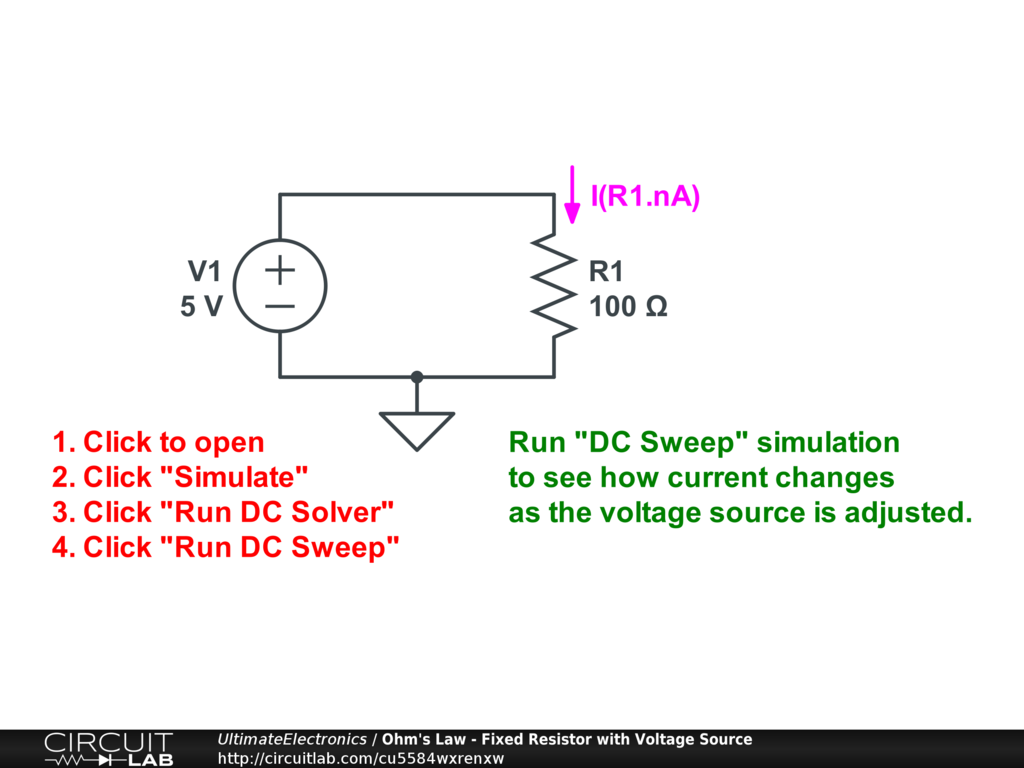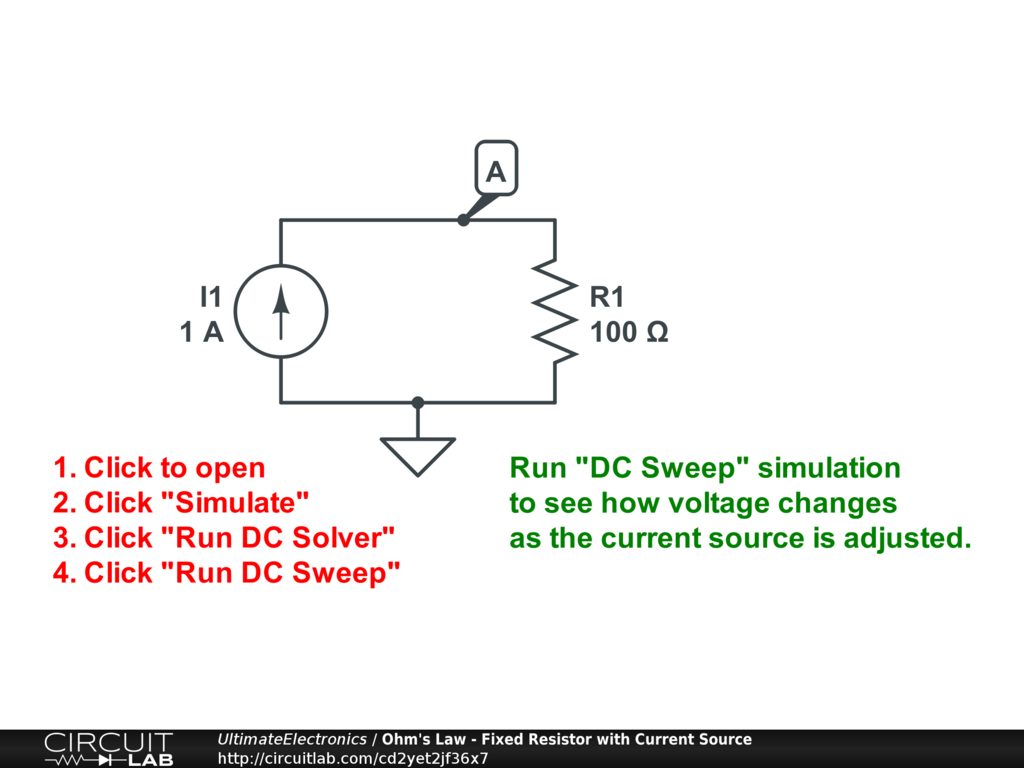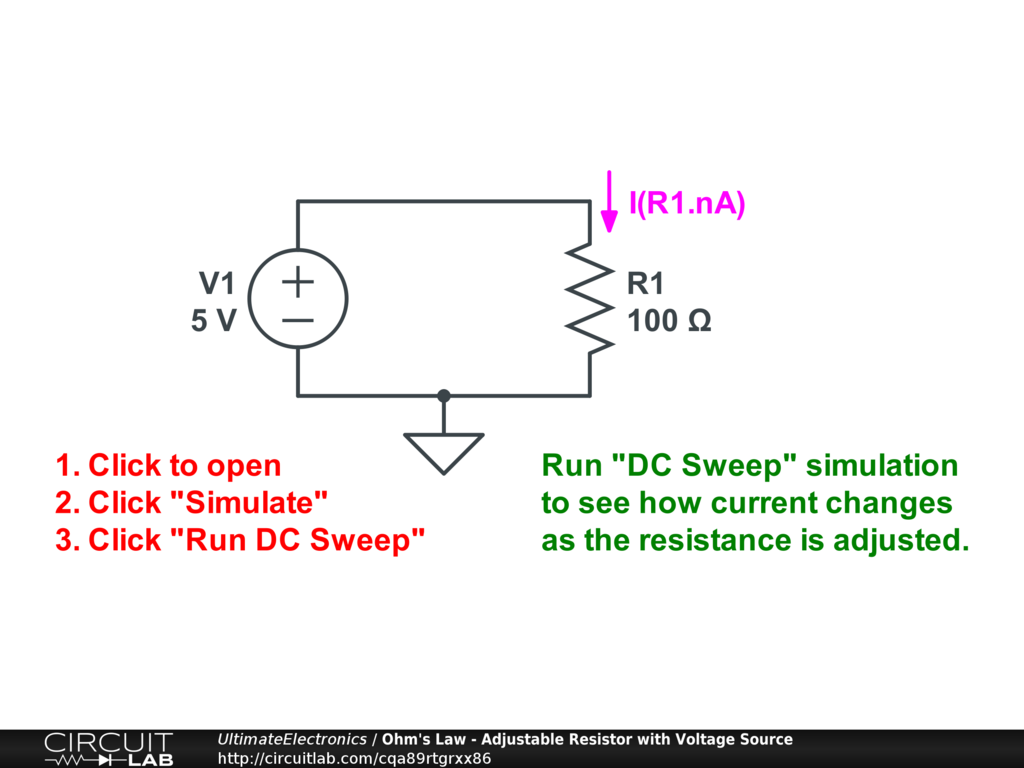← Prev: Ground
→ Next: Power

Resistance and Ohm's Law

In the Electrons in Motion section we discussed how electric fields cause a force on an electron. Just as with any object experiencing a net force, the electron will accelerate in response to that force. Over time, this acceleration causes velocity to increase. A velocity of charge carriers can be described as an electric current.

For free electrons flying around in a vacuum, that's the end of the story.

However, for electrons traveling in a material -- usually but not always a solid -- collisions between the electron and the material itself are an incredibly dominant factor in the electron's motion.

While the electron is still accelerated by the electric field, it often loses energy via collisions with the material. Each collision disperses the kinetic energy that the electron had built up into the material, which becomes heat.

As a result, to keep electrons moving through a material at a constant average speed (zero average acceleration), we still need to keep adding energy to overcome the kinetic energy lost in collisions. This property is called resistance.

Friction Analogy

Resistance due to collisions is analogous to mechanical friction.

Imagine a block sliding down an inclined ramp due to gravity. (For example, a coin sliding down the cover of a book that we've tilted up from level.) If there were no friction, the velocity of the block would keep increasing and increasing without limit as it went down the ramp. But in the real world, there are frictional collisions between the surfaces of the two materials, so the block reaches some steady state velocity. At this steady state velocity, the energy gained by moving down in the gravitational potential energy field equals the energy lost to friction. When we average over many of these microscopic collisions, it appears that the block neither accelerates nor decelerates, but instead continues moving along at a constant speed.

This is truly dissipating kinetic energy. If it weren't, then adding energy would cause continued acceleration, rather than just maintenance of a constant speed.

A fluid analogy is also reasonable. Pushing a liquid through a pipe requires energy (usually specified as a pressure difference) to maintain a constant speed (usually specified as a flow rate) because the flow is continuously losing energy due to drag from the inner walls of the pipe, as well as to viscous interactions between fluid molecules. These drag forces do dissipate as heat. (However, because water has such a high specific heat, we usually don't notice this temperature rise in everyday life.)

Ohm's Law

Ohm's Law is commonly seen as:

$$V = I R$$

where $R$ is the resistance of the resistor, $V$ is the voltage drop across the resistor, and $I$ is the current through the resistor. (See the remainder of this chapter, including Kirchhoff's Voltage Law (KVL) and Kirchhoff's Current Law (KCL), Labeling Voltages, Currents, and Nodes, and Solving Circuit Systems for more information about specifying the voltage and current correctly!)

Since voltage is a measure of work per unit charge, Ohm's Law means that for higher resistance materials, it takes more work to push the same flow of current through.

Ohm's Law states that resistance is a linear function, but this rule is not universal; in fact, it is empirical (based on observations, rather than theory). It is actually a linearized model of trillions or more of atomic-scale interactions within a material, and it turns out that on average, the aggregated behavior looks roughly linear.

Solving Ohm's Law Problems

If we know any two of $V,\ I,\ \text{or}\ R$, we can solve for the third variable:

\begin{align} V & = I \cdot R \\ I & = \frac {V} {R} \\ R & = \frac {V} {I} \end{align}

This is one of the most basic practical equations in electronics which is why we're emphasizing it in all its forms.

These relationships can be considered from a theoretical perspective in relating the currents and voltages in a circuit, but they also have practical implications:

• If we have a known current flowing through a known resistance, we can multiply the two to get a voltage: $V = I \cdot R$.
• If we have a known voltage across a known resistance, we can divide the two to get a current: $I = \frac {V} {R}$.
• Finally, if we have a known voltage and known current, we can divide the two to get a resistance: $R = \frac {V} {I}$.

In the mathematical sense, when we use the word known, we're contrasting it with the unknown variable that we're solving for. (See Systems of Equations.)

But in a practical sense, known can mean two slightly different things:

• "Known" can mean extrinsincally controlled or fixed. For example, if we have a current source which always outputs 2 Amps, then this current is known because it is being controlled and set to a particular value.
• "Known" can mean measured. For example, we may measure a voltage of 6 Volts across the output of the current source. This voltage is known because it is measured, rather than fixed by some process.

In most questions involving Ohm's Law, we combine the two types of "knowns." To combine the two examples above, if our $2\ \text{A}$ current source is connected to some unknown resistance, and we measure $6\ \text{V}$ across the output, we can determine that the unknown resistance is:

$$R = \frac {V} {I} = \frac {6} {2} = 3 \ \Omega$$

The linearity of Ohm's Law is extremely useful in making measurements. Resistors are components that linearly translate a voltage difference into a current and vice-versa, and this is a useful effect in many analog circuits. We'll talk more about voltmeters, ammeters, and ohmmeters in Multimeters & Measurements.

Ohm's Law Examples

Fixed Resistance with Voltage Source

Our first example is a single $100 \ \Omega$ resistor R1 connected to a voltage source:Interactive Exercise Click the circuit, then click "Simulate," and click "Run DC Solver." This will show the calculated current:

\begin{align} I & = \frac {V} {R} \\ & = \frac {5 \ \text{V}} {100 \ \Omega} \\ & = 0.05 \ \text{A} \\ & = 50 \ \text{mA} \end{align}

Then, click "Run DC Sweep." This is configured to adjust the value of the voltage source from -5 to +5. The simulator will instantly plot a graph with the voltage setting on the x-axis and the resulting current on the y-axis.

Fixed Resistance with Current Source

Instead of driving a resistance with a voltage source, we can instead connect it to a fixed current source:Interactive Exercise Click the circuit, click "Simulate," and "Run DC Solver." This will show the calculated voltage:

\begin{align} V_A & = I \cdot R \\ & = (1 \ \text{A}) \cdot (100 \ \Omega) \\ & = 100 \ \text{V} \end{align}

Next, click "Run DC Sweep." This is configured to adjust the value of the current source. The simulator will instantly plot a graph with the current setting on the x-axis, and the resulting voltage on the y-axis.

Using the CircuitLab simulator to vary currents and voltages and plot a DC Sweep graph is very basic functionality. A slightly more advanced case is to vary the resistance:Interactive Exercise Click the circuit, click "Simulate," and "Run DC Sweep." Now, the simulator plugs in different resistors from 1 to 1000 Ohms, and plots the resulting current curve, with resistance in Ohms on the x-axis, and current on the y-axis.

This works by setting the DC Sweep to vary "R1.R", which means "the resistance of the resistor named R1." Sweeping over a component's parameters is a powerful tool in circuit simulation.

Resistivity in Ohmic Materials

Resistance is a property of the choice of material (i.e. aluminum vs. copper), and its physical dimensions. For a solid of constant cross-sectional area $A$ and length $L$, the resistance is:

$$R = \rho \frac{L}{A}$$

The dimensions make sense: if we make a resistor twice as long, there is twice as much distance for collisions to take place and remove energy, just like resistors in series. Instead, if we increase the cross-sectional area, the average drift velocity is lower for the same amount of current, so less energy is lost in each collision.

The resistivity $\rho$ is a property of the material, and is also a function of temperature: see Pratical Resistors: Temperature Coefficient for more.

Why Resistivity is Linear

Why is current linearly proportional to voltage in a resistive material?

This is often taken for granted, but it's really not obvious. In free space, a charge in a constant electric field would have a linearly increasing velocity, rather than an (on average) constant velocity in a resistive material.

Here's one possible model:

Even with zero applied electric field (i.e. zero voltage), charges are not a rest. Due to thermal motion, they are constantly bumping around.

Now, let's apply an electric field (i.e. a nonzero voltage). This field $\vec{E}$ field causes a force on a charge:

$$\vec{F} = q \vec{E} = m \vec{a}$$

This force acts to accelerate the charge slightly in the direction of the field. However, before it gets going particularly fast, the charge collides with other charges within the material. This collision causes the loss of kinetic energy (converted to heat in the material), and the charge must start at zero velocity again. From there, it begins accelerating due to the field, and the cycle of acceleration and collision repeats.

Let's suppose that the average time between collisions is $t_{\text{collision}}$. In that case, in between collisions, the $\vec{E}$ field can accelerate a charge from $v=0$ to some velocity $v_{\text{max}}$ before collision. In this case (with fixed $t_{\text{collision}}$), the average velocity of the particle will be proportional to the acceleration due to the field. (If this is not clear, plot the velocity of the charge versus time. It will look like a sawtooth: increasing from 0 to $v_{\text{max}}$ at time $t_{\text{collision}}$, then suddenly dropping to 0 and repeating again. The average velocity is $\overline{v_d} = \frac {v_{\text{max}}} {2}$.)

For more detail on how this all plays out with random thermal vibrations in three dimensions, look up the Drude model.

You may wonder why we have an average time between collisions, rather than an average distance between collisions. This is because the thermal velocity is much much greater than the drift velocity, so these random thermal velocities basically determine when a collision happens, rather than the drift velocity being important in determining the collision rate.

For most materials, higher temperature means higher thermal velocities and shorter $t_{\text{collision}}$, so higher resistance. For more on the relationship between resistance and temperature, see Pratical Resistors: Temperature Coefficient.

Limits of Linearity

Resistors are typically linear over many orders of magnitude of current -- unlike a semiconductor junction, like we'll see later.

Resistors are typically linear regardless of which direction current is flowing -- also unlike a semiconductor junction.

Think of a mechanical coil spring: force is linear for tiny pushes and for big pushes, but at some point, you start to permanently deform the metal (plastic deformation) and you get nonlinear behavior.

In the same way, if you exceed the limits of the resistor, you may permanently change it, typically by overheating. See the next few sections on Power and Practical Resistors: Power Rating (Wattage) for more.

Superconductors

Superconductors were once exotic materials but are becoming more and more common as they come down in price and up in working temperature range. There are currently no known materials that are superconductors at room temperature and pressure, but research in this field has been finding materials that work at progressively higher and higher temperatures.

Superconductors are not just low resistance but offer truly zero resistance as current flows.

There are no internal inelastic collisions between charge carriers and material in a superconductor.

There are practical limits on superconductors in a few dimensions:

1. Current. A superconductor may only be able to support a certain maximum current density, known as the critical current.
2. Magnetic field. Superconducting materials have limits on the magnetic field strength they can operate in.
3. Operating temperature. Superconducting materials stop being superconducting above some maximum temperature limit.

In addition to these, superconductors and superconducting wire is still relatively complicated to work with from a manufacturing standpoint. The materials are often brittle, need to be cooled to extremely low temperatures, and are hard to join and connect to other components.

What's Next

In the next section, Power, we'll talk about where the heat from these resistive collisions goes, and think more about the flow of energy and power in general.